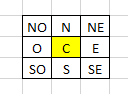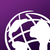317
3
09-04-2019 12:00 PMOccasional Contributor III

Hello everyone,

I dont know where to ask this, it is related to arcmap and python.

I have a layer that it is a grid (i think it is called fishnet) and I have several fields (Represented with C in the diagram with some value). I have to calculate the adjacent fields of C ( N, NE , NO , O, E, SO ,S , SE ) and their value too.

How can archieve that?I am pretty new using the tool and I want to know more or less the arctools that i have to use to get the values around the center.
There is another issue, sometimes not all the adjacent fields have value (I could be for example, NO or N are not available, but the rest of the other are).

Of course, every N, NE , NO , O, E, SO ,S , SE has their own value, and the logic that I have to apply is the following:
N = C(value) + N(value)
NE = C(value) + NE(Value)
SO = C(value) + SO (Value)
etc.

After that i have to only filter the values that have a certain range, so most likely not all the values that i will get are going to work.

I hope somebody can guide me on how to do this.

Tags (1)
3 RepliesbyMVP

This code works and does the calculations of the surrounding blocks then writes them to a CSV file that can be opened in excel. I only tested the script with a polygon fishnet layer. This might work with a polyline fishnet layer. Since I didn't know where you stored the number information to be calculated, for simplicity I assumed the number information would be stored in the fishnet layer as well. If you are working with two different layers please let me know so I can swap around some variables so the geometry function within the code checks for touches between the two layers and not within a single layer.

The code is also commented to help you change the needed variables to point to the correct places within your dataset. The script will also make a folder called Calculation in the same directory as the script, so you should be able to find it easily.

The variables you need to change are:

name_Field

number_Field

centerBlock

fishNetFc

``````import arcpy
import os
import csv

# This code is meant to run with python 3 which is the python version of ArcGIS Pro

########################################################################################################################
########################################################################################################################
#
#   CHANGE THE VARIABLES BELOW
#
########################################################################################################################
########################################################################################################################

# This variable is the name of the field that contains the label information for the Fishnet layer. Change it to match

name_Field = "Name"

# This variable is the name of the field that contains the number information that will be calculated. Change it to match

number_Field = "Number"

# This variable is the label name of the center block in the fishnet layer. Replace it with the name of the center block in your data

ceneterBlock = "C"

# This variable is the file path to the Fishnet layer. Change the text in between the quotes with the path to your fishnet layer
# Do not delete the r or the quotation marks

fishNetFC = r"C:\Users\JohnDoe\Documents\ArcGIS\Projects\General\General.gdb\Fishnet_test_polygon"

########################################################################################################################
########################################################################################################################
#
#      STOP, DO NOT CHANGE ANYTHING ELSE
#
########################################################################################################################
########################################################################################################################

# This creates the Calculation folder to store the CSV file if it doesnt exist.

if os.path.exists(os.path.dirname(os.path.abspath(__file__)) + "\\" + "Calculation") == False:
os.mkdir(os.path.dirname(os.path.abspath(__file__)) + "\\" + "Calculation")

# This deletes the old CSV file so the calculations are the most current run in the CSV file

if os.path.exists(os.path.dirname(os.path.abspath(__file__)) + "\\" + "Calculation" + "\\" + "FishNetCalc.csv") == True:
os.remove(os.path.dirname(os.path.abspath(__file__)) + "\\" + "Calculation" + "\\" + "FishNetCalc.csv")

# This is the path of where the CSV with the calculations will be created.

fishNetCalcCsv = os.path.dirname(os.path.abspath(__file__)) + "\\" + "Calculation" + "\\" + "FishNetCalc.csv"

# This variable creates and opens the CSV file as writable

csvWriter = open(fishNetCalcCsv, "w", newline="")

# This creates the headers in the CSV file
with csvWriter as f:
writerEdit = csv.writer(f)
writerEdit.writerow(["Center_Block", "Surrounding_Block", "Calculation"])

# This does a loop through the fishnet layer. Finds the center block, pauses at the middle block, does another loop through the data again to allow for calculations against
# the middle block
for geomTouchLoop1 in arcpy.da.SearchCursor(fishNetFC, ["SHAPE@", name_Field, number_Field]):
if geomTouchLoop1 == ceneterBlock:
for geomTouchLoop2 in arcpy.da.SearchCursor(fishNetFC, ["SHAPE@", name_Field, number_Field]):
if geomTouchLoop2 == ceneterBlock:
continue
# This if statement does a geometry check on all of the geometry that touches the middle block then does the calculations and writes them to the CSV file
if geomTouchLoop1.touches(geomTouchLoop2):
writer2 = open(fishNetCalcCsv, "a", newline="")
with writer2 as f2:
writer2Edit = csv.writer(f2)
calculations = geomTouchLoop1 + geomTouchLoop2
writer2Edit.writerow([geomTouchLoop1, geomTouchLoop2, calculations])

print("Done!!")
exit()‍‍‍‍‍‍‍‍‍‍‍‍‍‍‍‍‍‍‍‍‍‍‍‍‍‍‍‍‍‍‍‍‍‍‍‍‍‍‍‍‍‍‍‍‍‍‍‍‍‍‍‍‍‍‍‍‍‍‍‍‍‍‍‍‍‍‍‍‍‍‍‍‍‍‍‍‍‍‍‍‍``````Occasional Contributor

I updated the code to for the calculation to handle exceptions for non-integer entries. The code also kicks out text to let you know where it found a non-integer and it will pass over it doing the calculations for the other blocks.

It will say something like this:

Found a Non-Integer In the fishnet layer, at block: SO Continuing
Doing Calculation for block: C and Block: S
Doing Calculation for block: C and Block: SE
Found a Non-Integer In the fishnet layer, at block: O Continuing
Doing Calculation for block: C and Block: E
Doing Calculation for block: C and Block: NO
Doing Calculation for block: C and Block: N
Found a Non-Integer In the fishnet layer, at block: NE Continuing
Done!!

Here is the updated code:

``````import arcpy
import os
import csv

# This code is meant to run with python 3 which is the python version of ArcGIS Pro

########################################################################################################################
########################################################################################################################
#
#   CHANGE THE VARIABLES BELOW
#
########################################################################################################################
########################################################################################################################

# This variable is the name of the field that contains the label information for the Fishnet layer. Change it to match

name_Field = "Name"

# This variable is the name of the field that contains the number information that will be calculated. Change it to match

number_Field = "Number"

# This variable is the label name of the center block in the fishnet layer. Replace it with the name of the center block in your data

ceneterBlock = "C"

# This variable is the file path to the Fishnet layer. Change the text in between the quotes with the path to your fishnet layer
# Do not delete the r or the quotation marks

fishNetFC = r"C:\Users\JohnDoe\Documents\ArcGIS\Projects\General\General.gdb\Fishnet_test_polygon"

########################################################################################################################
########################################################################################################################
#
#      STOP, DO NOT CHANGE ANYTHING ELSE
#
########################################################################################################################
########################################################################################################################

# This creates the Calculation folder to store the CSV file if it doesnt exist.

if os.path.exists(os.path.dirname(os.path.abspath(__file__)) + "\\" + "Calculation") == False:
os.mkdir(os.path.dirname(os.path.abspath(__file__)) + "\\" + "Calculation")

# This deletes the old CSV file so the calculations are the most current run in the CSV file

if os.path.exists(os.path.dirname(os.path.abspath(__file__)) + "\\" + "Calculation" + "\\" + "FishNetCalc.csv") == True:
os.remove(os.path.dirname(os.path.abspath(__file__)) + "\\" + "Calculation" + "\\" + "FishNetCalc.csv")

# This is the path of where the CSV with the calculations will be created.

fishNetCalcCsv = os.path.dirname(os.path.abspath(__file__)) + "\\" + "Calculation" + "\\" + "FishNetCalc.csv"

# This variable creates and opens the CSV file as writable

csvWriter = open(fishNetCalcCsv, "w", newline="")

# This creates the headers in the CSV file
with csvWriter as f:
writerEdit = csv.writer(f)
writerEdit.writerow(["Center_Block", "Surrounding_Block", "Calculation"])

# This does a loop through the fishnet layer. Finds the center block, pauses at the middle block, does another loop through the data again to allow for calculations against
# the middle block
for geomTouchLoop1 in arcpy.da.SearchCursor(fishNetFC, ["SHAPE@", name_Field, number_Field]):
if geomTouchLoop1 == ceneterBlock:
for geomTouchLoop2 in arcpy.da.SearchCursor(fishNetFC, ["SHAPE@", name_Field, number_Field]):
if geomTouchLoop2 == ceneterBlock:
continue
# This if statement does a geometry check on all of the geometry that touches the middle block then does the calculations and writes them to the CSV file
if geomTouchLoop1.touches(geomTouchLoop2):
writer2 = open(fishNetCalcCsv, "a", newline="")
with writer2 as f2:
writer2Edit = csv.writer(f2)
try:
calculations = int(geomTouchLoop1) + int(geomTouchLoop2)
writer2Edit.writerow([geomTouchLoop1, geomTouchLoop2, calculations])
print("Doing Calculation for block:" + " " + geomTouchLoop1 + " " + "and" + " " + "Block:" + " " + geomTouchLoop2)
except:
print("Found a Non-Integer In the fishnet layer, at block:" + " " + geomTouchLoop2 + " " + "Continuing")
#writer2Edit.writerow([geomTouchLoop1, geomTouchLoop2, calculations])

print("Done!!")
exit()‍‍‍‍‍‍‍‍‍‍‍‍‍‍‍‍‍‍‍‍‍‍‍‍‍‍‍‍‍‍‍‍‍‍‍‍‍‍‍‍‍‍‍‍‍‍‍‍‍‍‍‍‍‍‍‍‍‍‍‍‍‍‍‍‍‍‍‍‍‍‍‍‍‍‍‍‍‍‍‍‍‍‍‍‍‍``````Occasional Contributor III

Thanks very much! I will try it.